# How to split text string in Excel by comma, space, character or mask

The tutorial explains how to split cells in Excel using formulas and the Split Text feature. You will learn how to separate text by comma, space or any other delimiter, and how to split strings into text and numbers.

Splitting text from one cell into several cells is the task all Excel users are dealing with once in a while. In one of our earlier articles, we discussed how to split cells in Excel using the Text to Column feature, Flash Fill and Split Names add-in. Today, we are going to take an in-depth look at how you can split strings using formulas and the Split Text feature.

## How to split text in Excel using formulas

To split string in Excel, you generally use the LEFT, RIGHT or MID function in combination with either FIND or SEARCH. At first sight, some of the formulas might look complex, but the logic is in fact quite simple, and the following examples will give you some clues.

### Split string by comma, colon, slash, dash or other delimiter

When splitting cells in Excel, the key is to locate the position of the delimiter within the text string. Depending on your task, this can be done by using either case-insensitive SEARCH or case-sensitive FIND. Once you have the delimiter's position, use the RIGHT, LEFT or MID function to extract the corresponding part of the text string. For better understanding, let's consider the following example.

Supposing you have a list of SKUs of the Item-Color-Size pattern, and you want to split the column into 3 separate columns: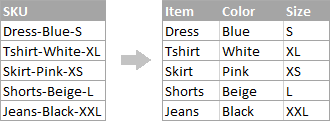1. To extract the item name (all characters before the 1st hyphen), insert the following formula in B2, and then copy it down the column:

`=LEFT(A2, SEARCH("-",A2,1)-1)`

In this formula, SEARCH determines the position of the 1st hyphen ("-") in the string, and the LEFT function extracts all the characters left to it (you subtract 1 from the hyphen's position because you don't want to extract the hyphen itself).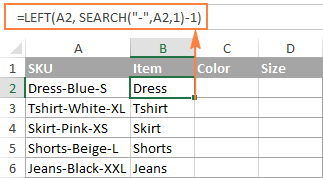2. To extract the color (all characters between the 1st and 2nd hyphens), enter the following formula in C2, and then copy it down to other cells:

`=MID(A2, SEARCH("-",A2) + 1, SEARCH("-",A2,SEARCH("-",A2)+1) - SEARCH("-",A2) - 1)`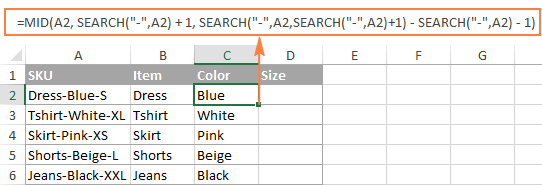In this formula, we are using the Excel MID function to extract text from A2.

The starting position and the number of characters to be extracted are calculated with the help of 4 different SEARCH functions:

• Start number is the position of the first hyphen +1:

`SEARCH("-",A2) + 1`

• Number of characters to extract: the difference between the position of the 2nd hyphen and the 1st hyphen, minus 1:

`SEARCH("-", A2, SEARCH("-",A2)+1) - SEARCH("-",A2) -1`

3. To extract the size (all characters after the 3rd hyphen), enter the following formula in D2:

`=RIGHT(A2,LEN(A2) - SEARCH("-", A2, SEARCH("-", A2) + 1))`

In this formula, the LEN function returns the total length of the string, from which you subtract the position of the 2nd hyphen. The difference is the number of characters after the 2nd hyphen, and the RIGHT function extracts them.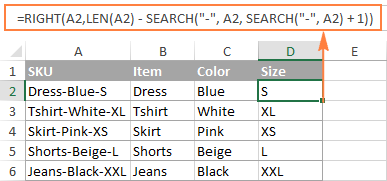In a similar fashion, you can split column by any other character. All you have to do is to replace "-" with the required delimiter, for example space (" "), slash ("/"), colon (";"), semicolon (";"), and so on.

Tip. In the above formulas, +1 and -1 correspond to the number of characters in the delimiter. In this example, it's a hyphen (1 character). If your delimiter consists of 2 characters, e.g. a comma and a space, then supply only the comma (",") to the SEARCH function, and use +2 and -2 instead of +1 and -1.

### How to split string by line break in Excel

To split text by space, use formulas similar to the ones demonstrated in the previous example. The only difference is that you will need the CHAR function to supply the line break character since you cannot type it directly in the formula.

Supposing, the cells you want to split look similar to this: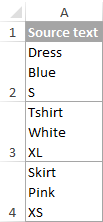Take the formulas from the previous example and replace a hyphen ("-") with CHAR(10) where 10 is the ASCII code for Line feed.

• To extract the item name:

`=LEFT(A2, SEARCH(CHAR(10),A2,1)-1)`

• To extract the color:

`=MID(A2, SEARCH(CHAR(10),A2) + 1, SEARCH(CHAR(10),A2,SEARCH(CHAR(10),A2)+1) - SEARCH(CHAR(10),A2) - 1)`

• To extract the size:

`=RIGHT(A2,LEN(A2) - SEARCH(CHAR(10), A2, SEARCH(CHAR(10), A2) + 1))`

And this is how the result looks like: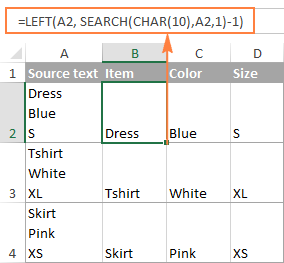### How to split text and numbers in Excel

To begin with, there is no universal solution that would work for all alphanumeric strings. Which formula to use depends on the particular string pattern. Below you will find the formulas for the two common scenarios.

### Split string of 'text + number' pattern

Supposing, you have a column of strings with text and numbers combined, where a number always follows text. You want to break the original strings so that the text and numbers appear in separate cells, like this: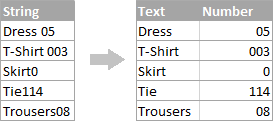The result may be achieved in two different ways.

#### Method 1: Count digits and extract that many chars

The easiest way to split text string where number comes after text is this:

To extract numbers, you search the string for every possible number from 0 to 9, get the numbers total, and return that many characters from the end of the string.

With the original string in A2, the formula goes as follows:

`=RIGHT(A2,SUM(LEN(A2) - LEN(SUBSTITUTE(A2, {"0","1","2","3","4","5","6","7","8","9"},""))))`

To extract text, you calculate how many text characters the string contains by subtracting the number of extracted digits (C2) from the total length of the original string in A2. After that, you use the LEFT function to return that many characters from the beginning of the string.

`=LEFT(A2,LEN(A2)-LEN(C2))`

Where A2 is the original string, and C2 is the extracted number, as shown in the screenshot: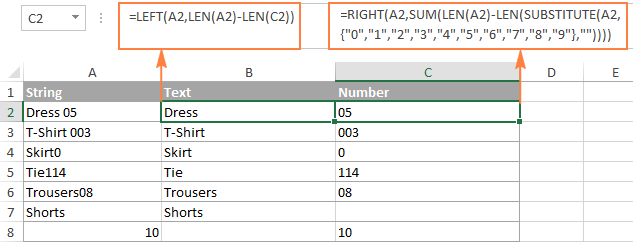#### Method 2: Find out the position of the 1st digit in a string

An alternative solution would be using the following formula to determine the position of the first digit in the string:

`=MIN(SEARCH({0,1,2,3,4,5,6,7,8,9},A2&"0123456789"))`

The detailed explanation of the formula's logic can be found here.

Once the position of the first digit is found, you can split text and numbers by using very simple LEFT and RIGHT formulas.

To extract text:

`=LEFT(A2, B2-1)`

To extract number:

`=RIGHT(A2, LEN(A2)-B2+1)`

Where A2 is the original string, and B2 is the position of the first number.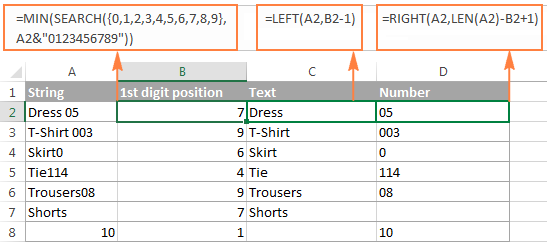To get rid of the helper column holding the position of the first digit, you can embed the MIN formula into the LEFT and RIGHT functions:

Formula to extract text:

`=LEFT(A2,MIN(SEARCH({0,1,2,3,4,5,6,7,8,9},A2&"0123456789"))-1)`

Formula to extract numbers:

`=RIGHT(A2,LEN(A2)-MIN(SEARCH({0,1,2,3,4,5,6,7,8,9},A2&"0123456789"))+1)`

### Split string of 'number + text' pattern

If you are splitting cells where text appears after number, you can extract numbers with the following formula:

`=LEFT(A2, SUM(LEN(A2) - LEN(SUBSTITUTE(A2, {"0","1","2","3","4","5","6","7","8","9"}, ""))))`

The formula is similar to the one discussed in the previous example, except that you use the LEFT function instead of RIGHT to get the number from the left side of the string.

Once you have the numbers, extract text by subtracting the number of digits from the total length of the original string:

`=RIGHT(A2,LEN(A2)-LEN(B2))`

Where A2 is the original string and B2 is the extracted number, as shown in the screenshot below: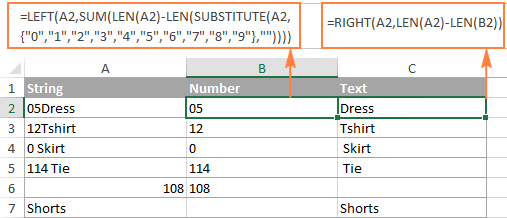Tip. To get number from any position in the text string, use either this formula or the Extract tool.

This is how you can split strings in Excel using different combinations of different functions. As you see, the formulas are far from obvious, so you may want to download the sample Excel Split Cells workbook to examine them closer.

If figuring out the arcane twists of Excel formulas is not your favorite occupation, you may like the visual method to split cells in Excel, which is demonstrated in the next part of this tutorial.

## How to split cells in Excel with the Split Text feature

An alternative way to split a column in Excel is using the Split Text feature included with our Ultimate Suite for Excel, which provides the following options:

To make things clearer, let's have a closer look at each option, one at a time.

### Split cells by character

Choose this option whenever you want to split the cell contents at each occurrence of the specified character.

For this example, let's the take the strings of the Item-Color-Size pattern that we used in the first part of this tutorial. As you may remember, we separated them into 3 different columns using 3 different formulas. And here's how you can achieve the same result in 2 quick steps:

1. Assuming you have Ultimate Suite installed, select the cells to split, and click the Split Text icon on the Ablebits Data tab.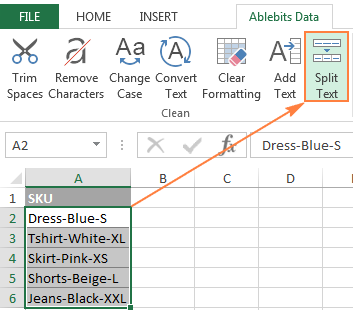2. The Split Text pane will open on the right side of your Excel window, and you do the following:
• Expand the Split by character group, and select one of the predefined delimiters or type any other character in the Custom box.
• Choose whether to split cells to columns or rows.
• Review the result under the Preview section, and click the Split button.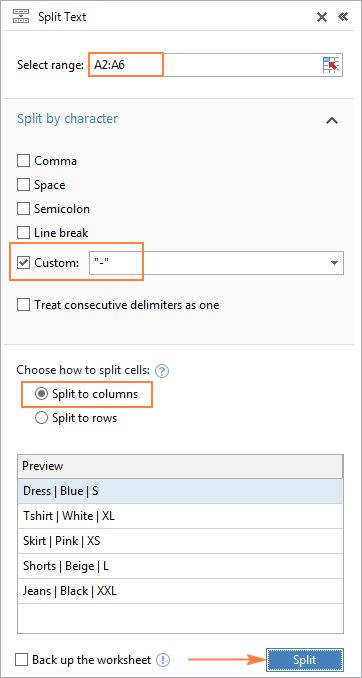Tip. If there might be several successive delimiters in a cell (for example, more than one space character), select the Treat consecutive delimiters as one box.

Done! The task that required 3 formulas and 5 different functions now only takes a couple of seconds and a button click.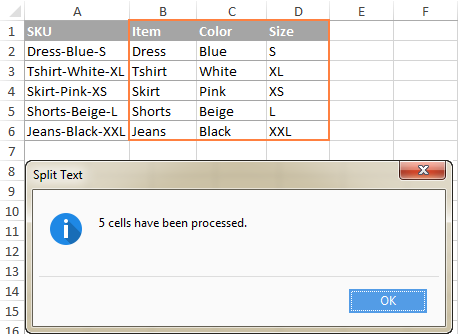### Split cells by string

This option lets you split strings using any combination of characters as a delimiter. Technically, you split a string into parts by using one or several different substrings as the boundaries of each part.

For example, to split a sentence by the conjunctions "and" and "or", expand the Split by strings group, and enter the delimiter strings, one per line: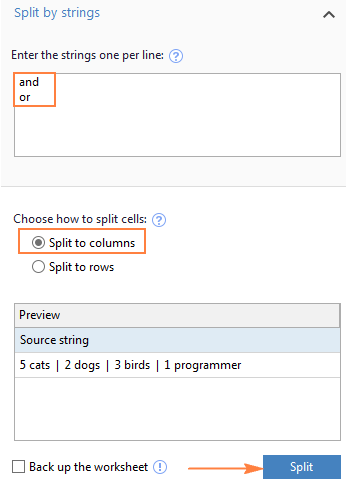As the result, the source phrase is separated at each occurrence of each delimiter: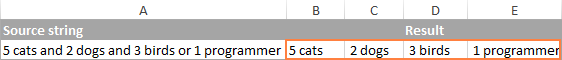Tip. The characters "or" as well as "and" can often be part of words like "orange" or "Andalusia", so be sure to type a space before and after and and or to prevent splitting words.

And here another, real-life example. Supposing you've imported a column of dates from an external source, which look as follows:

5.1.2016 12:20
5.2.2016 14:50

This format is not conventional for Excel, and therefore none of the Date functions would recognize any of the date or time elements. To split day, month, year, hours and minutes into separate cells, enter the following characters in the Split by strings box:

• Dot (.) to separate day, month, and year
• Colon (:) to separate hours and minutes
• Space to separate date and time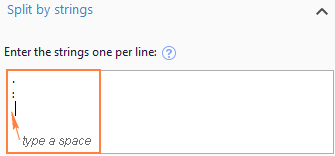Hit the Split button, and you will immediately get the result: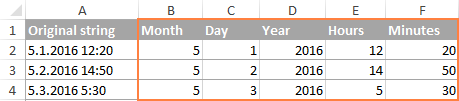### Split cells by mask (pattern)

Separating a cell by mask means splitting a string based on a pattern.

This option comes in very handy when you need to split a list of homogeneous strings into some elements, or substrings. The complication is that the source text cannot be split at each occurrence of a given delimiter, only at some specific occurrence(s). The following example will make things easier to understand.

Supposing you have a list of strings extracted from some log file: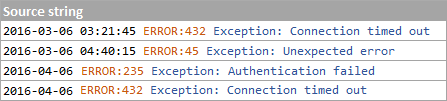What you want is to have date and time, if any, error code and exception details in 3 separate columns. You cannot utilize a space as the delimiter because there are spaces between date and time, which should appear in one column, and there are spaces within the exception text, which should also appear in one column.

The solution is splitting a string by the following mask: *ERROR:*Exception:*

Where the asterisk (*) represents any number of characters.

The colons (:) are included in the delimiters because we don't want them to appear in the resulting cells.

And now, expand the Split by mask section on the Split Text pane, type the mask in the Enter delimiters box, and click Split: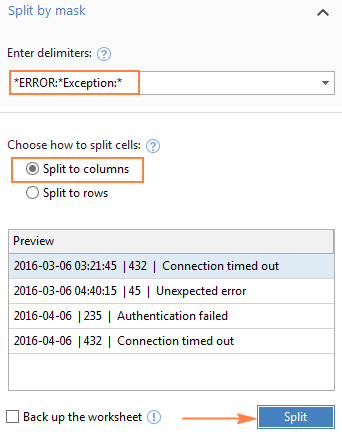The result will look similar to this: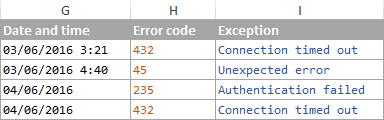Note. Splitting string by mask is case-sensitive. So, be sure to type the characters in the mask exactly as they appear in the source strings.

A big advantage of this method is flexibility. For example, if all of the original strings have date and time values, and you want them to appear in different columns, use this mask:

* *ERROR:*Exception:*

Translated into plain English, the mask instructs the add-in to divide the original strings into 4 parts:

• All characters before the 1st space found within the string (date)
• Characters between the 1st space and the word ERROR: (time)
• Text between ERROR: and Exception: (error code)
• Everything that comes after Exception: (exception text)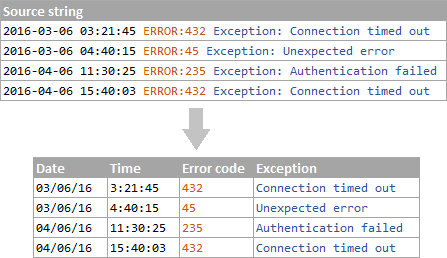I hope you liked this quick and straightforward way to split strings in Excel. If you are curious to give it a try, an evaluation version is available for download below. I thank you for reading and hope to see you on our blog next week!